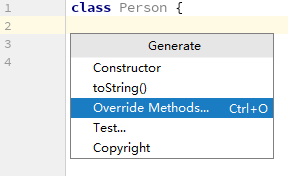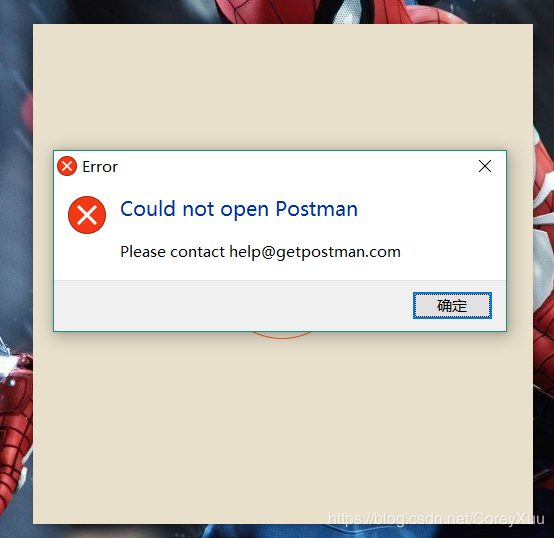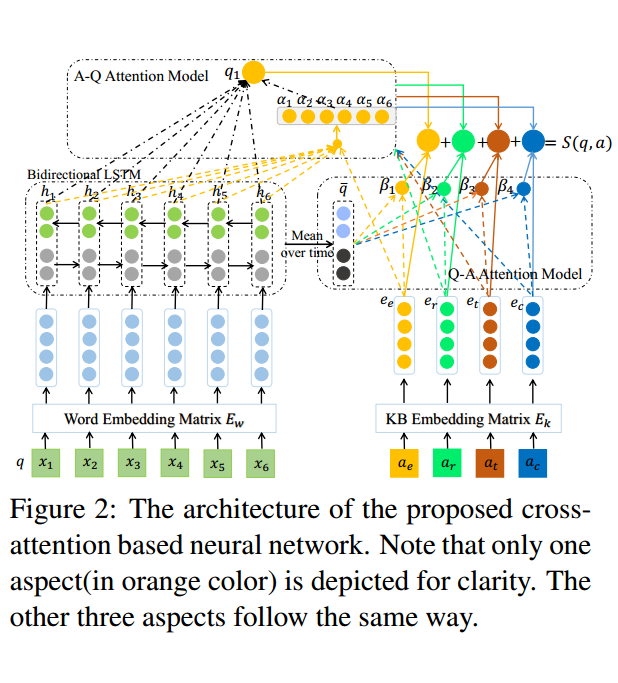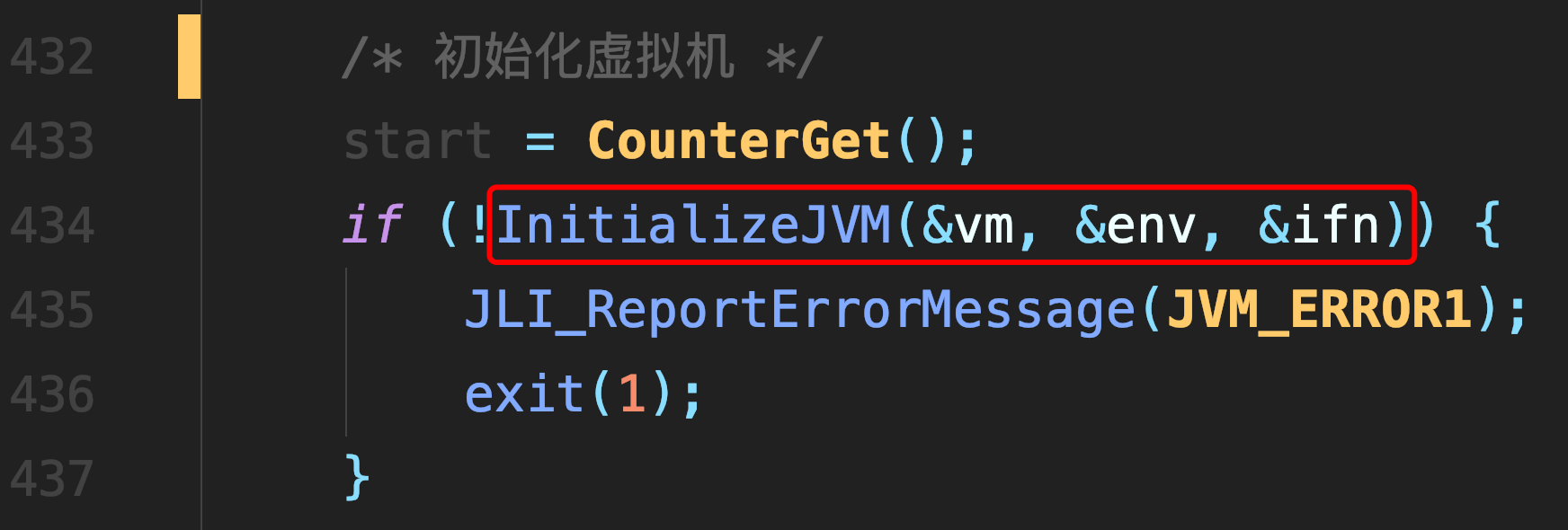# 并查集：刷题必备神器

## 并查集：刷题必备神器

### 并查集的初始化``````//并查集最大容纳元素个数
class UFS{
public int []arr;
//存储各个集合元素
public int [] number;
public UFS(int MAX_SIZE) {
public static int MAX_SIZE = 10000;
public static int arr[] = new int[MAX_SIZE];
//存储每个集合元素数量
public static int number[] = new int[MAX_SIZE];
}
public void init(){
for(int i = 0; i < arr.length; i++) arr[i] = i;
for(int i = 0; i < arr.length; i++) number[i] = 1;
}
}
``````

### 并查集的查询操作``````//中间有路径压缩过程，可以细细分析一下
public int find(int x){
if(x != arr[x]) arr[x] = find(arr[x]);
return arr[x];
}
``````

### 并查集的合并操作``````public void union(int x, int y) {
int xRoot = find(x);
int yRoot = find(y);
if(xRoot == yRoot) return;
if(arr[xRoot] > arr[yRoot]) {
arr[xRoot] += arr[yRoot];
arr[yRoot] = xRoot;
}else{
arr[yRoot] += arr[xRoot];
arr[xRoot] = yRoot;
}
}
``````

### 参考文章

##### 热门推荐
•浏览(655)
•浏览(565)
•浏览(548)
•浏览(488)
•浏览(433)
•浏览(424)
•浏览(381)
•浏览(367)
•浏览(362)
•浏览(357)